# 哈希表

## 介绍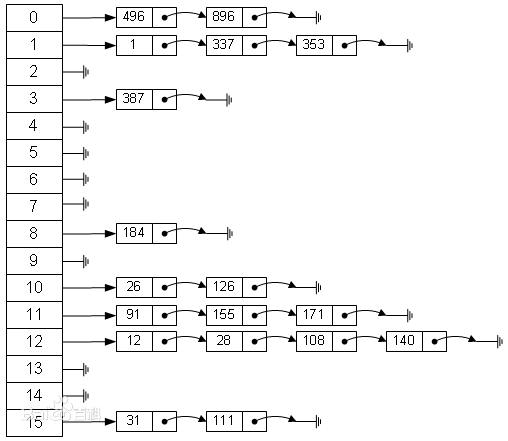## 简易实现Hash表

### Node节点编写

class Emp {
public int id;
public String name;
public Emp next; // next默认为空

public Emp(int id, String name) {
this.id = id;
this.name = name;
}
}


### 实现链表

class EmpLinkedList {
}


public void add(Emp emp) {
// 如果是添加第一个雇员
return;
}
// 如果不是第一个雇员则需要一个辅助的指针帮助定位到最后
while (cur.next != null) {
cur = cur.next;
}
// 退出时候将emp加入到最后即可
cur.next = emp;
}


#### 遍历list方法

// 遍历链表的雇员信息
public void list(int no) {
System.out.println("第" + no + "链表为空");
return;
}
System.out.print("第" + no + "链表的信息为：");
while (true) {
if (cur == null) {
return;
}
System.out.printf("->id=%d name=%s\t", cur.id, cur.name);
cur = cur.next;
}
}


#### 查找方法

// 根据id查找雇员
public Emp findEmpById(int id) {
// 判断链表是否为空
return null;
}
while (true) {
if(cur.id == id) {
break;
}
if(cur.next == null) {
cur = null;
break;
}
cur = cur.next;
}
return cur;
}


### HashTab实现

// 创建hashTable
class HashTab {
private int size;

// 构造器
public HashTab(int size) {
this.size = size;
// 初始化链表
// 实例化每一个链表
for (int i = 0; i < size; i++) {
}
}
}


#### 散列方法编写

// 编写一个散列函数，使用简单的取模法
public int hashFun(int id) {
return id % size;
}


#### 添加方法编写

public void add(Emp emp) {
// 首先根据员工的id，得到该员工应该添加到那条链表
// 将emp添加到对应的链表中
}


#### 遍历方法编写

// 遍历哈希表
public void list() {
for (int i = 0; i < size; i++) {
System.out.println();
}
}


#### 查找方法编写

// 查找雇员
public void findEmpById(int id) {
// 使用散列函数到那条链表查找
if (emp != null) {
} else {
System.out.println("未找到");
}
}


### 测试代码

public class HashTableDemo {
public static void main(String[] args) {
HashTab h = new HashTab(5);
Emp e1 = new Emp(1, "张三");
Emp e2 = new Emp(2, "李四");
Emp e3 = new Emp(3, "王五");
Emp e4 = new Emp(4, "赵六");
Emp e5 = new Emp(5, "钱七");
Emp e6 = new Emp(6, "魏八");
h.list();
h.findEmpById(5);
}
}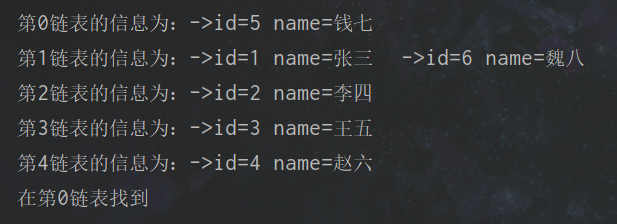# 树(二叉树)

## 基本概念介绍(树)

### 基本形态

1. 空二叉树
2. 只有一个根节点的二叉树
3. 只有左子树
4. 只有右子树
5. 完全二叉树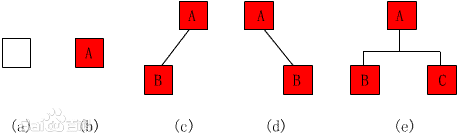### 术语介绍

• 节点：包含一个数据元素及若干指向子树分支的信息
• 节点的度：一个结点拥有子树的数目称为结点的度
• 叶子节点：也称为终端结点，没有子树的结点或者度为零的结点
• 层次：根节点为第1层，一次类推，根节点的子节点为第2层
• 树的高度：当前树的最大层次，即为树的高度
• 森林：简单来说就是由多个不相交的树就能构成一个森林

### 二叉树的性质

1. 二叉树的第i层上至多有$2^{i+1}$ (i>=1)个节点
2. 深度为h的二叉树中之多含有$2^h-1$个节点
3. 若在任意一颗二叉树中，有$n_1$个叶子节点，有$n_2$个度为2的节点，则必有$n_1=n_2+1$
4. 具有n个节点的完全二叉树高度为$log_2(n-1)+1$个节点

## 二叉树的遍历

### 前序/中序/后序遍历的实现

#### 思想

1. 前序遍历

1. 中序遍历

1. 后序遍历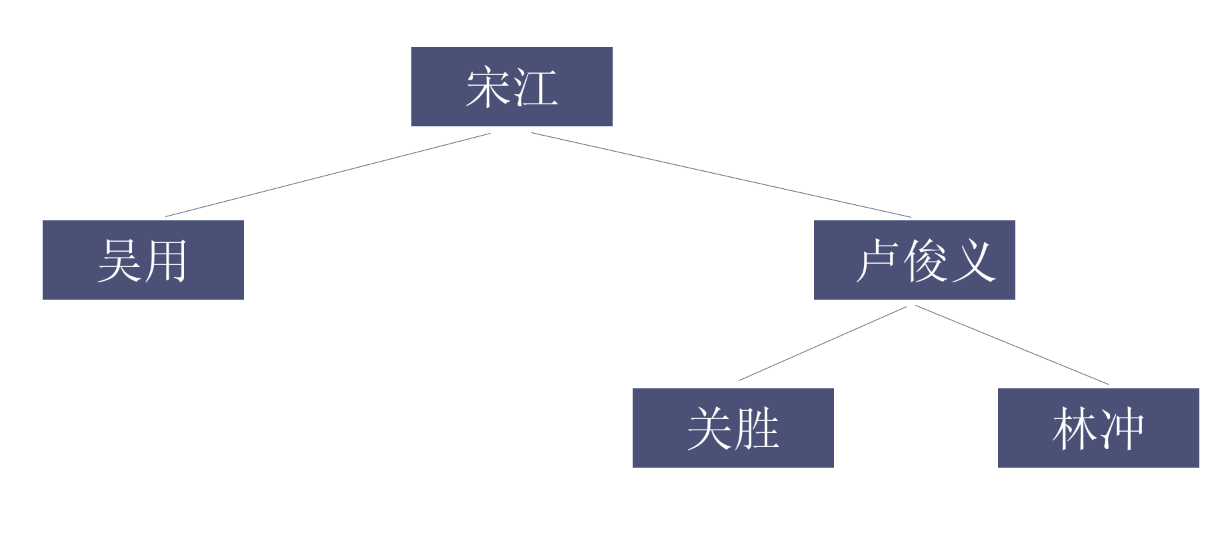#### 实现

class HeroNode {
private int no;
private String name;
private HeroNode left; // 默认null
private HeroNode right; // 默认null

public HeroNode(int no, String name) {
this.no = no;
this.name = name;
}
// 略写了Get和Set方法
@Override
public String toString() {
return "HeroNode{" +
"no=" + no +
", name='" + name + '\'' +
'}';
}
// 前序遍历的方法
public void preOrder() {
System.out.println(this); // 先输出父节点
// 递归向左子树
if (this.left != null) {
this.left.preOrder();
}
// 递归向右子树
if (this.right != null) {
this.right.preOrder();
}
}
// 中序遍历
public void infixOrder() {
// 递归向左子树
if (this.left != null) {
this.left.infixOrder();
}
System.out.println(this);
// 递归向右子树
if (this.right != null) {
this.right.infixOrder();
}
}
// 后序遍历
public void postOrder() {
if (this.left != null) {
this.left.postOrder();
}
if (this.right != null) {
this.right.postOrder();
}
System.out.println(this);
}
}


// 定义一个BinaryTree二叉树
class BinaryTree {
private HeroNode root;

public void setRoot(HeroNode root) {
this.root = root;
}

// 前序遍历
public void preOrder() {
if (this.root != null) {
this.root.preOrder();
} else {
System.out.println("二叉树为空");
}
}

// 中序遍历
public void infixOrder() {
if (this.root != null) {
this.root.infixOrder();
} else {
System.out.println("二叉树为空");
}
}

// 后序遍历
public void postOrder() {
if (this.root != null) {
this.root.postOrder();
} else {
System.out.println("二叉树为空");
}
}
}


public class BinaryTreeDemo {
public static void main(String[] args) {
// 创建一棵二叉树
BinaryTree binaryTree = new BinaryTree();
// 创建节点
HeroNode root = new HeroNode(1, "宋江");
HeroNode node2 = new HeroNode(2, "吴用");
HeroNode node3 = new HeroNode(3, "卢俊义");
HeroNode node4 = new HeroNode(4, "林冲");
binaryTree.setRoot(root);

// 说明，我们先手动创建二叉树
root.setLeft(node2);
root.setRight(node3);
node3.setRight(node4);
System.out.println("前序遍历");
binaryTree.preOrder();
System.out.println("中序遍历");
binaryTree.infixOrder();
System.out.println("后序遍历");
binaryTree.postOrder();
}
}


## 二叉树的节点删除

### 规定

1. 如果待删除的节点为叶子节点，则直接删除该节点
2. 如果待删除的节点不是叶子节点，则删除节点代表的子树

### 思想分析

1. 因为我们的二叉树是单向的，所以我们要找的是待删除节点的父节点(类似于单向链表的删除)
2. 如果当前节点的左子节点，不为空且左子节点是待删除节点，就删除该节点或者该子树(this.left=null)且递归结束
3. 如果当前节点的右子节点，不为空且右子节点是待删除节点，就删除该节点或者该子树(this.right=null)且递归结束
4. 如果第2步和第3步没有删除节点，那么我们需要向左子树进行递归删除
5. 如果第4步也没有删除节点，则需要向右子树进行递归删除

### 代码实现

public void delNode(int no) {
if (this.left != null && this.left.no == no) {
this.left = null;
return;
}
if (this.right != null && this.right.no == no) {
this.right = null;
return;
}
// 向左子树进行递归删除
if (this.left != null) {
this.left.delNode(no);
}
// 向右子树进行递归删除
if (this.right != null) {
this.right.delNode(no);
}
}


// 删除节点
public void delNode(int no) {
if (root != null) {
// 如果只有一个root节点，这里要判断root是否为待删除节点
if (root.getNo() == no) {
root = null;
} else {
// 进行递归删除
root.delNode(no);
}
} else {
System.out.println("空树不可以删除");
}
}


### 测试代码

public class BinaryTreeDemo {
public static void main(String[] args) {
// 创建一棵二叉树
BinaryTree binaryTree = new BinaryTree();
// 创建节点
HeroNode root = new HeroNode(1, "宋江");
HeroNode node2 = new HeroNode(2, "吴用");
HeroNode node3 = new HeroNode(3, "卢俊义");
HeroNode node4 = new HeroNode(4, "林冲");
binaryTree.setRoot(root);

// 说明，我们先手动创建二叉树
root.setLeft(node2);
root.setRight(node3);
node3.setRight(node4);
System.out.println("删除前的前序遍历");
binaryTree.preOrder();
binaryTree.delNode(4);
System.out.println("-------");
System.out.println("删除后的前序遍历");
binaryTree.preOrder();
}
}


## 顺序存储二叉树

### 介绍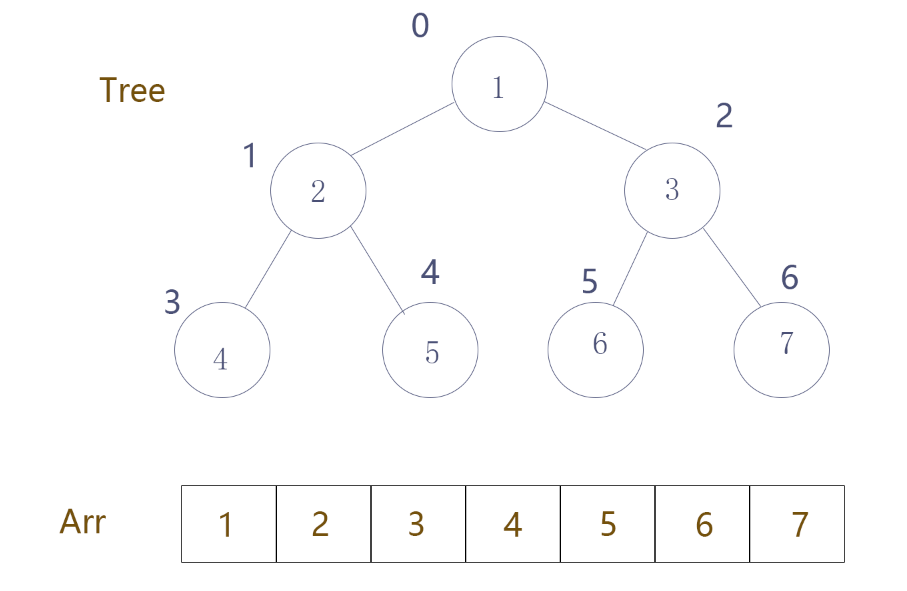### 性质

1. 顺序存储二叉树，通常情况只考虑完全二叉树
2. 第n个元素的左子节点索引为 2 * n + 1
3. 第n个元素的右子节点索引为 2 * n + 2
4. 第n个元素的父节点索引为 (n - 1) / 2
5. 上述n代表的是在顺序存储二叉树中是第几个元素，也可以理解为数组的下标，在上述图中也就是圆圈外的数字

### 代码实现案例

class ArrBinaryTree {
private int[] arr; // 用于存储二叉树

public ArrBinaryTree(int[] arr) {
this.arr = arr;
}

// 暴露重载的方法不用输入索引简化操作
public void preOrder() {
this.preOrder(0);
}

// 编写方法用于遍历顺序存储的二叉树的前序遍历

public void preOrder(int i) {
// 数组为空或者arr.length=0
if (arr == null || arr.length == 0) {
System.out.println("数组为空无法遍历！");
}
// 首先打印本元素
System.out.print(arr[i]);
// 对左子树进行前序遍历，注意判断公式不能超出数组边界
if ((i * 2 + 1) < arr.length) {
preOrder(i * 2 + 1);
}

// 对右子树进行前序遍历，注意判断公式不能超出数组边界
if ((i * 2 + 2) < arr.length) {
preOrder(i * 2 + 2);
}
}
}


public class ArrBinaryTreeDemo {
public static void main(String[] args) {
int[] arr = {1, 2, 3, 4, 5, 6, 7};
ArrBinaryTree abt = new ArrBinaryTree(arr);
abt.preOrder();
}
}


## 线索化二叉树

### 线索化二叉树介绍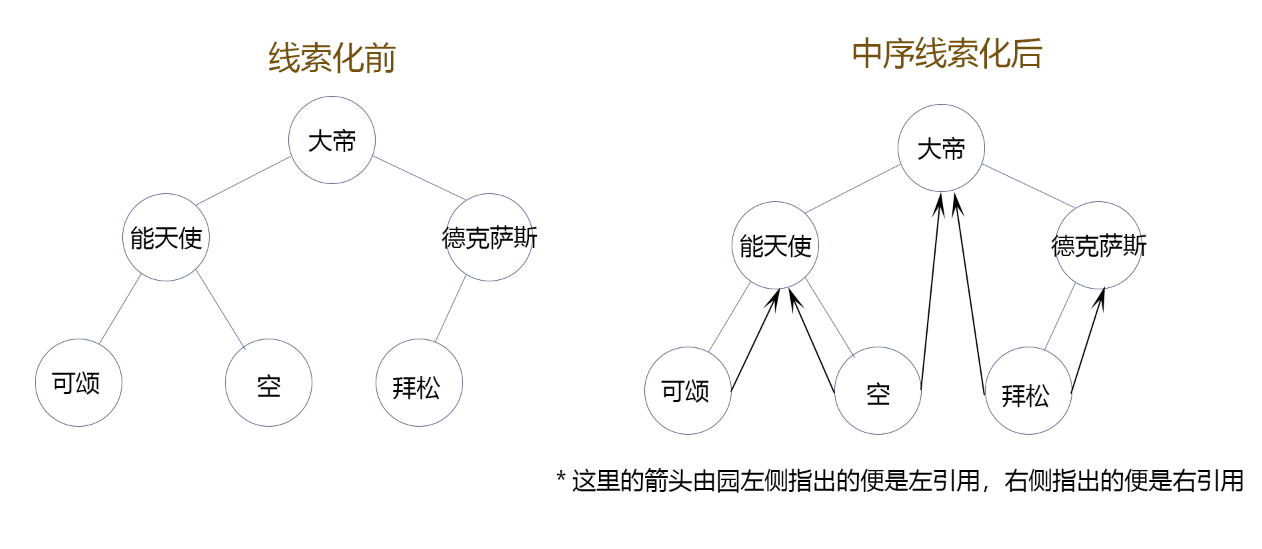### 线索二叉树的实现

// 说明：
// 如果leftType=0表示指向的是左子树如果是1表示指向前驱节点
// rightType同理
private int leftType;
private int rightType;


// 为了实现线索化需要创建一个指向当前节点的前驱节点的一个指针
// 在递归进行线索化时，这个pre总是保留前一个节点
private HeroNode pre = null;


// 编写对二叉树进行中序线索化的方法
// 如果当前线索化的节点为空就不能线索化
if (node == null) {
return;
}
// 1. 先线索化左子树
// 2. 线索化当前节点[有点难度]

// 处理当前节点的前驱节点
if (node.getLeft() == null) {
// 让当前节点的左指针指向前驱节点
node.setLeft(pre);
// 修改当前节点的左指针的类型
node.setLeftType(1); // 指向的时前驱节点
}
// 处理后继节点
if (pre != null && pre.getRight() == null) {
// 让前驱节点的右指针指向当前节点
pre.setRight(node);
// 修改前驱节点的右指针类型
pre.setRightType(1);
}
// !!! 每处理一个节点后，让当前节点时下一个节点的前驱节点
pre = node;

// 3. 线索化右子树
}


### 小作业

#### 图示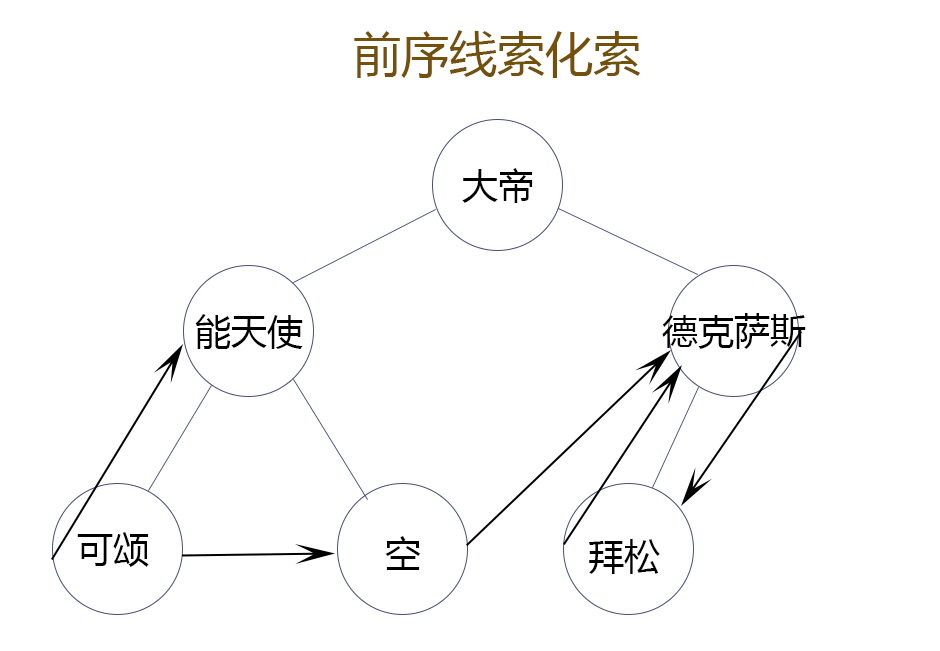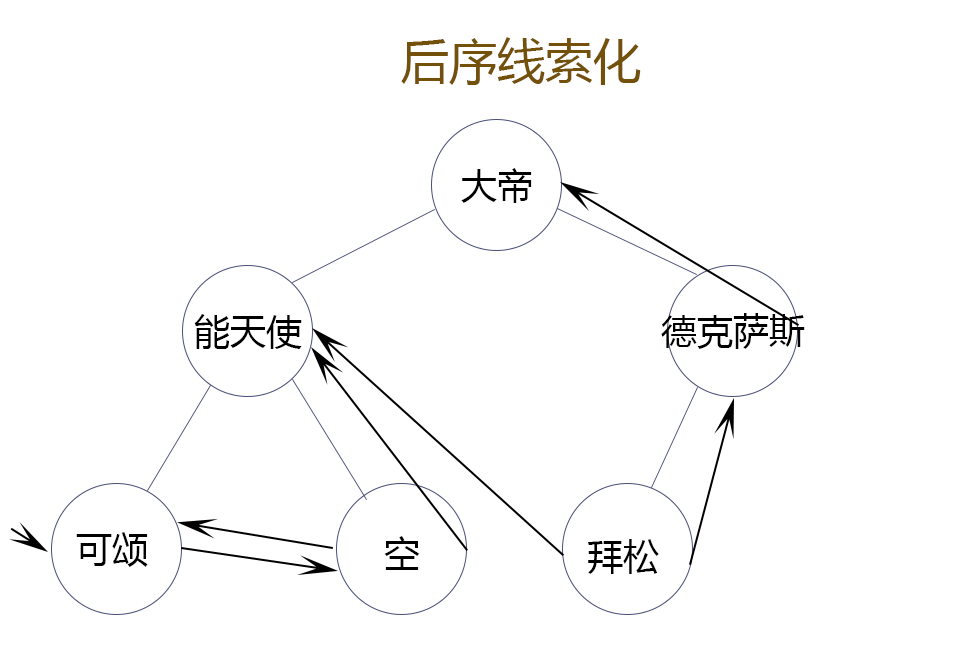#### 实现代码

public void preThreadedNodes(HeroNode node) {
if (node == null) {
return;
}

// 1. 线索化当前节点
// 处理当前节点的前驱节点
if (node.getLeft() == null) {
// 让当前节点的左指针指向前驱节点
node.setLeft(pre);
// 修改当前节点的左指针的类型
node.setLeftType(1); // 指向的时前驱节点
}
// 处理后继节点
if (pre != null && pre.getRight() == null) {
// 让前驱节点的右指针指向当前节点
pre.setRight(node);
// 修改前驱节点的右指针类型
pre.setRightType(1);
}
// !!! 每处理一个节点后，让当前节点时下一个节点的前驱节点
pre = node;
// 2. 线索化左子树
if (node.getLeftType() == 0) {
}
// 3. 线索化右子树
if (node.getRightType() == 0) {
}
}


public void postThreadedNodes(HeroNode node) {
if (node == null) {
return;
}
// 1. 线索化左子树
// 2. 线索化右子树
// 3. 线索化当前节点
// 处理当前节点的前驱节点
if (node.getLeft() == null) {
// 让当前节点的左指针指向前驱节点
node.setLeft(pre);
// 修改当前节点的左指针的类型
node.setLeftType(1); // 指向的时前驱节点
}
// 处理后继节点
if (pre != null && pre.getRight() == null) {
// 让前驱节点的右指针指向当前节点
pre.setRight(node);
// 修改前驱节点的右指针类型
pre.setRightType(1);
}
// !!! 每处理一个节点后，让当前节点时下一个节点的前驱节点
pre = node;
}


### 线索化二叉树的遍历

ps 实现后两种遍历方式(尤其是后序的，真的很难)，参考了很多网上大佬写的文章。

// 中序线索化二叉树遍历方法一
// 定义一个变量，存储当前遍历的节点，从root开始
HeroNode node = root;
while(node != null) {
while (node.getLeftType() == 0) {
node = node.getLeft();
}
System.out.println(node);
// 如果当前节点的右指针指向的是后继节点，就一直输出
while (node.getRightType() == 1) {
node = node.getRight();
System.out.println(node);
}
// 替换这个遍历的节点
node = node.getRight();
}
}


1. 首先，从root节点开始往左进行查找，直到当前节点也就是node的左面存储的不再是左子树而是存储的线索。这也是第一个内循环做的事情。
2. 打印当前node
3. 再查看当前节点的右面存储的是否是线索，是的话就把线索指向的节点作为当前节点打印后再查看是否为线索
4. 如果右面不是线索，也就是说右面指向的是一个子树，就把当前节点变为这个子树，外循环结束，如果这个子树不为空，就继续执行外循环，直到下次外循环的这个子树为空，外循环结束，就遍历完成。

public void threadedList2() {
HeroNode node = root;
while(node != null) {
if(node.getLeftType() == 0) {
node = node.getLeft();

}else {
System.out.println(node);
if(node.getRightType() == 1) {
node = node.getRight();
System.out.println(node);
}
node = node.getRight();
}
}
}


1. 首先我认为，这两种算法都是用的一个内核，也就是一个思想，只是在小操作上不同
2. 虽然我也看不出在实际应用中那个更胜，但是就我浅显的来看我更喜欢第二种，这样写看似少写了两个循环但是在循环次数上应该和第一种差不多，因为它把第一种的3个循环集成在了一个上，每一句代码都利用到了极致，真的是写的精彩。

public void threadedListBefore() {
// 定义一个变量，存储当前遍历的节点，从root开始
HeroNode node = root;
while (node != null) {
while (node.getLeftType() == 0) {
System.out.println(node);
node = node.getLeft();
}
System.out.println(node);
node = node.getRight();
}
}


// 父节点 后序线索化遍历时使用
private HeroNode parent;


// 后序线索化二叉树遍历
//1、找后序遍历方式开始的节点
HeroNode node = root;
while ( node != null && node.getLeftType() == 0 ) {
node = node.getLeft();
}
while ( node != null ) {
//右节点是线索
if (node.getRightType() == 1) {
System.out.println(node);
pre = node;
node = node.getRight();
} else {
//如果上个处理的节点是当前节点的右节点
if (node.getRight() == pre) {
System.out.println(node);
if (node == root) {
return;
}
pre = node;
node = node.getParent();
} else {
//如果从左节点的进入则找到有子树的最左节点
node = node.getRight();
while ( node != null && node.getLeftType() == 0 ) {
node = node.getLeft();
}
}
}
}
}


BinaryTree binaryTree = new BinaryTree();
binaryTree.setRoot(root);